Rota, Gian-Carlo

Rota's basis conjecture ★★★

Author(s): Rota

Conjecture   Let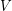be a vector space of dimensionand let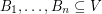be bases. Then there existdisjoint transversals ofeach of which is a base.

Keywords: base; latin square; linear algebra; matroid; transversal

Rota's unimodal conjecture ★★★

Author(s): Rota

Let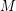be a matroid of rank, and for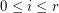let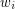be the number of closed sets of rank.

Conjectureis unimodal.
Conjectureis log-concave.

Keywords: flat; log-concave; matroid